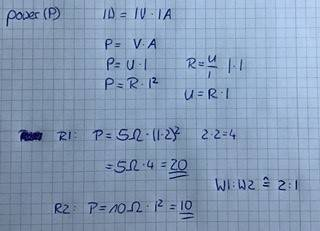# Thermal energy/ heat in a parallel connection

AnneX
Homework Statement:
Nope, I am studying for a quiz
Relevant Equations:
Not sure, but R and W could be directly proportional...
In a parallel connection heat is produced.

R1 = 5 Ohm

R2 = 10 Ohm

What is the relation of W1/W2?

1:4

1:2

2:1

1:8

3:1

I’d tend to say 1:2, but I am not really sure…

Homework Helper
Gold Member
Whats the formula for W? (When you know R and you know something else because they are in parallel.)

AnneX
The current flowing through R1 is double the current flowing through R2

Homework Helper
Gold Member
Do you know any formula for the heat generated W in a resistance R when a current I flows and there is a pd of V across it?

Homework Helper
Gold Member
Or, actually, you've <nearly> solved it with your correct answer ##I_1 = 2 I_2##

Edited in <>

Homework Helper
Gold Member
Do you know
Power=Voltage x Current ##W=VI##
Voltage = Current x Resistance ##V=IR##
So ## W= VI \ = \ IR I \ = \ I^2R##
or ## I = \frac{V}{R} ##
So ## W= VI \ = \ V \frac{V}{R} \ = \ \frac{V^2}{R} ##

•AnneX
AnneXSo, it is 2:1?

Homework Helper
Gold Member
Yes.
You can always put some numbers in, as you do here, but the formulae are useful to remember.

Important thing here is, to know that the voltage is the same across both, when they in parallel.
So P=IV tells you that power is proportional to current. Current 2:1 so power 2:1

If they were in series, the current would be the same. If you work out the voltages,
P=IV tells you the power is proportional to the voltage for the same current.

•AnneX
AnneX
Thanks for the help 👍
One more question though, if the two resistors were in series would it be W1 : W2 = 1:2

Last edited:
Homework Helper
Gold Member
One more question though, if the two resistors were in series would it be W1 : W2 = 1:2
Yes.

•AnneX